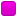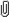June 04, 2023, 03:31:55 am

###AuthorTopic: MATH1041 or MATH1141  (Read 5228 times) Tweet Share

0 Members and 1 Guest are viewing this topic.

#### Gli1

•• Posts: 6
• Respect: 0##### MATH1041 or MATH1141
« on: December 29, 2020, 10:03:33 am »
0
Hi everyone,
Ive recently enrolled into adv science at unsw, majoring in pharmacology. Ive also achieved a HSC mark of 99 in extension 1 and 96 in extension 2 maths.

From the course structure recommendations, they recommended MATH1041 (statistics for life and social sciences), which from what I understand is just for people studying sciences to get maths out of the way.

But since I achieved a pretty decent combined mark of 195 in maths courses in the HSC, I feel like doing MATH1041 is just a waste for my extension 2 knowledge, so instead I chose MATH1141.

What would you guys recommend me to do? Change from MATH1141 to MATH1041, remain my Enrolment as it is, or just do both (I.e 1 in term one and the other in term three?)

#### RuiAce

• ATAR Notes Lecturer
• Honorary Moderator
• Great Wonder of ATAR Notes
••• Posts: 8814
• "All models are wrong, but some are useful."
• Respect: +2575##### Re: MATH1041 or MATH1141
« Reply #1 on: December 29, 2020, 12:24:16 pm »
+6
The first thing to note is that MATH1041 and MATH1141 are substantially different from one another.

The actual 'get maths out of the way' is MATH1031. This course actually shares some overlap with the regular MATH1131/MATH1231 progression. The content is taught at a much lower level in contrast to MATH1131/MATH1231, and also with less depth. It's not required for many degrees either.

MATH1041 is a course taught at roughly the same difficulty as MATH1031. But as the course name suggests, the focus is on statistics. It only really assumes mathematical knowledge up to HSC Maths Standard 2, since you don't even have to do calculus in this course (at most they show the integral symbol but never use it). (Which is pretty much all the maths you need to know to learn statistics, provided that you're not interested in the theory behind it all.)

In my opinion, more degrees recommend MATH1041 over MATH1031 simply because they anticipate students to find the basic stats knowledge more useful. However, the statistics content is completely separate from the other maths-focused courses. In fact, MATH1041 is more equivalent to MATH2801/MATH2901, but with basically all the theory taken out. (Will note that some degrees do require students to do both anyway.)

On the other hand, MATH1131 is the more natural continuation of maths, and MATH1141 being its higher counterpart. The former assumes knowledge equivalent to HSC Maths Ext 1. The latter is only recommended if you've succeeded well in Ext 1 + Ext 2, which clearly you have. (Some infographics here and here, but these won't matter to you.)

During COVID, the difference between these two courses kinda got blurred. I don't know if there was any significant difference between the two, since they had to do online exams. Prior to COVID though, MATH1131 and MATH1141 were completely identical, up until the final exam. Half of the final exam is identical between MATH1131 and MATH1141, but as for the other half, MATH1141 do receive some notably more difficult problems. By taking MATH1141, you're basically saying "sure, come at me" to these problems. I think in the past, you were rewarded with some scaling for taking it over MATH1131.
____________________________________________________________________________________

With that out of the way, what should you be picking? For starters, I can confirm according to this UNSW handbook entry that you can take either-or. You also have room for electives as per this UNSW handbook entry should you want both. Specifically, 1 science elective or 1 free elective can be used if you want to do both.

Whilst statistics is often highly recommended for its usefulness, a university degree should also have elements of fun in it. (Otherwise you're just gonna hate your time.) So you might want to first ask this question: will you miss maths? If yes, then at the very least MATH1131 would be a good idea. And if you do enjoy the stimulation of maths, then MATH1141 is likely the better option.

Judging by that you feel MATH1041 might 'waste' your Ext 2 knowledge, you probably are headed down that road. But just ask yourself the question again if you're sure about it. If you really don't have the passion for maths anymore, then MATH1141 could backfire. But if you do, then MATH1141 could be one of the best decisions you make.

I can't really give my own opinion without being biased, since I literally study advanced maths at uni. And consequently would've just said do both regardless of the circumstances. Having said that though, doing both does have its perks. It feeds into any passion for math you have, whilst sacrificing just 1 mere elective to get the usefulness out of the statistics side. The only thing you need to accept is that MATH1041 is literally just a basic statistics course, without any intense maths at all.#### Gli1

•• Posts: 6
• Respect: 0##### Re: MATH1041 or MATH1141
« Reply #2 on: December 31, 2020, 10:26:06 pm »
0
Thank you for the detailed response!

Also, considering that you do advanced math, can you pls help with this q?
*from unsw vector booklet*

#### fun_jirachi

• MOTM: AUG 18
• HSC Moderator
• Part of the furniture
••• Posts: 1068
• All doom and Gloom.
• Respect: +710##### Re: MATH1041 or MATH1141
« Reply #3 on: December 31, 2020, 11:48:50 pm »
+3
Since $e_1, e_2, e_3, e_4$ is the standard basis for $\mathbb{R}^4$ they are perpendicular to each other as well as being linearly independent. Since the span of the two planes takes a different pair from the set ${e_1, e_2, e_3, e_4}$ for each plane, we already know that they aren't parallel. Solving simultaneously to find a point of intersection, we have that a vector that satisfies the equations of both planes must satisfy $s_1e_1 + (s_2-t_1)e_2 -t_2e_3 -e_4 = 0$, but since we established that ${e_1, e_2, e_3, e_4}$ are linearly independent (due to being a basis for $\mathbb{R}^4$), the only solution that exists must be the one where all coefficients are zero, which is impossible, since the coefficient on $e_4$ is set to -1. Hence, the planes do not interesect either.

If you need any more clarification lmkSpoiler
HSC 2018: Mod Hist  | 2U Maths 
HSC 2019: Physics  | Chemistry  | English Adv  | 3U Maths  | 4U Maths 
ATAR: 99.05

UCAT: 3310 - VR  | DM  | QR  | AR 
Subject Acceleration (2018)
UCAT Question Compilation/FAQ (2020)

#### RuiAce

• ATAR Notes Lecturer
• Honorary Moderator
• Great Wonder of ATAR Notes
••• Posts: 8814
• "All models are wrong, but some are useful."
• Respect: +2575##### Re: MATH1041 or MATH1141
« Reply #4 on: January 01, 2021, 01:53:31 am »
+4
Thank you for the detailed response!

Also, considering that you do advanced math, can you pls help with this q?
*from unsw vector booklet*Remark: You'd definitely get away with far more briefer explanations in an exam. Slightly less formality should also be acceptable for an assignment.

Since $e_1, e_2, e_3, e_4$ is the standard basis for $\mathbb{R}^4$ they are perpendicular to each other as well as being linearly independent. Since the span of the two planes takes a different pair from the set ${e_1, e_2, e_3, e_4}$ for each plane, we already know that they aren't parallel. Solving simultaneously to find a point of intersection, we have that a vector that satisfies the equations of both planes must satisfy $s_1e_1 + (s_2-t_1)e_2 -t_2e_3 -e_4 = 0$, but since we established that ${e_1, e_2, e_3, e_4}$ are linearly independent (due to being a basis for $\mathbb{R}^4$), the only solution that exists must be the one where all coefficients are zero, which is impossible, since the coefficient on $e_4$ is set to -1. Hence, the planes do not interesect either.

If you need any more clarification lmkYeah, linear independence gives a more direct shortcut to this question. Main problem is that it's more of a MATH1231/1241 technique; MATH1131/1141 students aren't expected to know linear independence. So I just went by a more first-principles approach.#### Gli1

•• Posts: 6
• Respect: 0##### Re: MATH1041 or MATH1141
« Reply #5 on: January 01, 2021, 08:09:30 am »
0
Can you pls elaborate on the span bit for explaining parallel?

#### RuiAce

• ATAR Notes Lecturer
• Honorary Moderator
• Great Wonder of ATAR Notes
••• Posts: 8814
• "All models are wrong, but some are useful."
• Respect: +2575##### Re: MATH1041 or MATH1141
« Reply #6 on: January 01, 2021, 11:45:57 am »
0
What about the span bit specifically?#### Gli1

•• Posts: 6
• Respect: 0##### Re: MATH1041 or MATH1141
« Reply #7 on: January 01, 2021, 07:46:21 pm »
0
What about the span bit specifically?

The entire section regarding the non-parallel nature of the plane. The whole span concept that the solution shows is a bit vague to me.

#### RuiAce

• ATAR Notes Lecturer
• Honorary Moderator
• Great Wonder of ATAR Notes
••• Posts: 8814
• "All models are wrong, but some are useful."
• Respect: +2575##### Re: MATH1041 or MATH1141
« Reply #8 on: January 01, 2021, 08:56:41 pm »
+2
The entire section regarding the non-parallel nature of the plane. The whole span concept that the solution shows is a bit vague to me.
It is just using the definition of the span given in the course notes.
$\operatorname{span}(\mathbf{v}_1, \mathbf{v}_2) = \{ \mathbf{x} : \mathbf{x} = \lambda_1 \mathbf{v}_1 + \lambda_2 \mathbf{v}_2\text{ for some }\lambda_1, \lambda_2 \in \mathbb R \}.$
Or is there something you don't understand within this definition? And/Or something specific to how it is being applied?#### Gli1

•• Posts: 6
• Respect: 0##### Re: MATH1041 or MATH1141
« Reply #9 on: January 01, 2021, 10:17:28 pm »
0
It is just using the definition of the span given in the course notes.
$\operatorname{span}(\mathbf{v}_1, \mathbf{v}_2) = \{ \mathbf{x} : \mathbf{x} = \lambda_1 \mathbf{v}_1 + \lambda_2 \mathbf{v}_2\text{ for some }\lambda_1, \lambda_2 \in \mathbb R \}.$
Or is there something you don't understand within this definition? And/Or something specific to how it is being applied?

I dont  get how that span definition is applied here in this q (I.e. the non-parallel proof in this q)

#### fun_jirachi

• MOTM: AUG 18
• HSC Moderator
• Part of the furniture
••• Posts: 1068
• All doom and Gloom.
• Respect: +710##### Re: MATH1041 or MATH1141
« Reply #10 on: January 01, 2021, 10:59:17 pm »
+1
If you reread the question, it basically says in the second line planes in $\mathbb{R}^n$ of the form $a + bv_1 + cv_2$ for real constants a, b, c and vectors in $\mathbb{R}^n$ are parallel if their spans are equal.

Essentially, they prove that the spans of each of the planes cannot possibly be equal by counterexample - they choose one vector in the span of the first plane, and note that it doesn't belong to the span of the second plane. Span is effectively an infinite set of vectors defined by the plane, and to prove that the planes are parallel, we have to prove set equality. This cannot and does not exist in this case, and hence the planes are not parallel.

Hope this clarifies things a bitSpoiler
HSC 2018: Mod Hist  | 2U Maths 
HSC 2019: Physics  | Chemistry  | English Adv  | 3U Maths  | 4U Maths 
ATAR: 99.05

UCAT: 3310 - VR  | DM  | QR  | AR